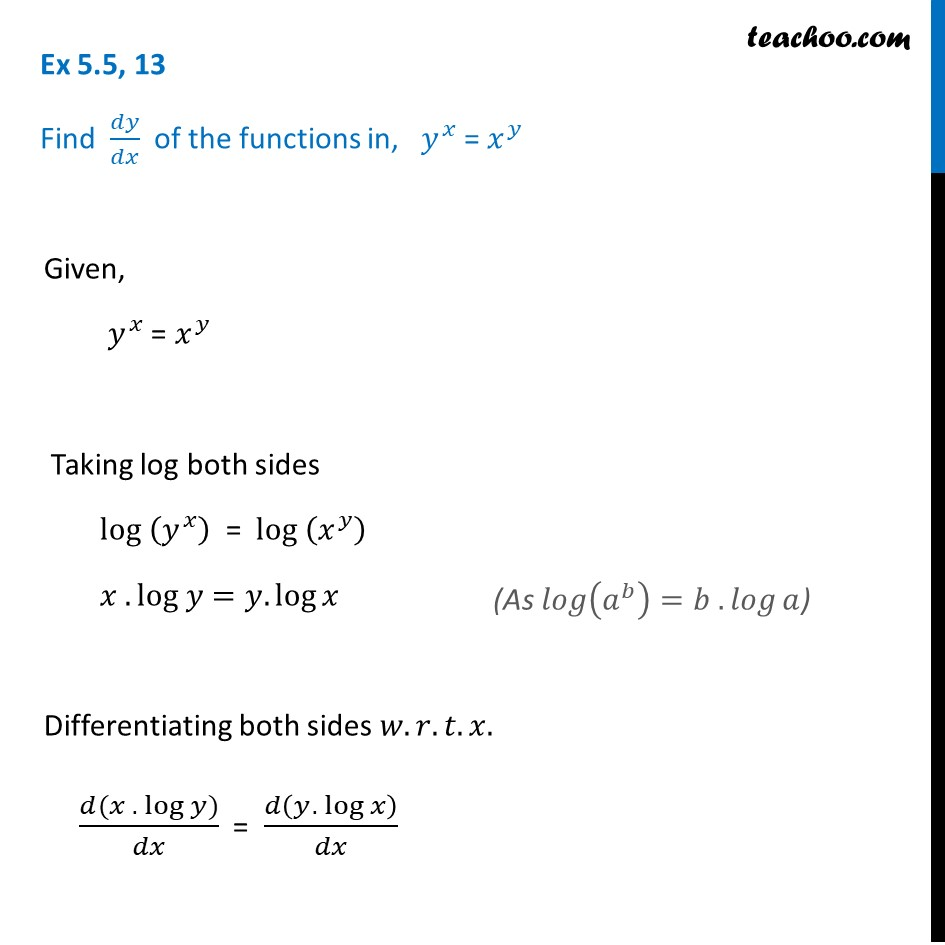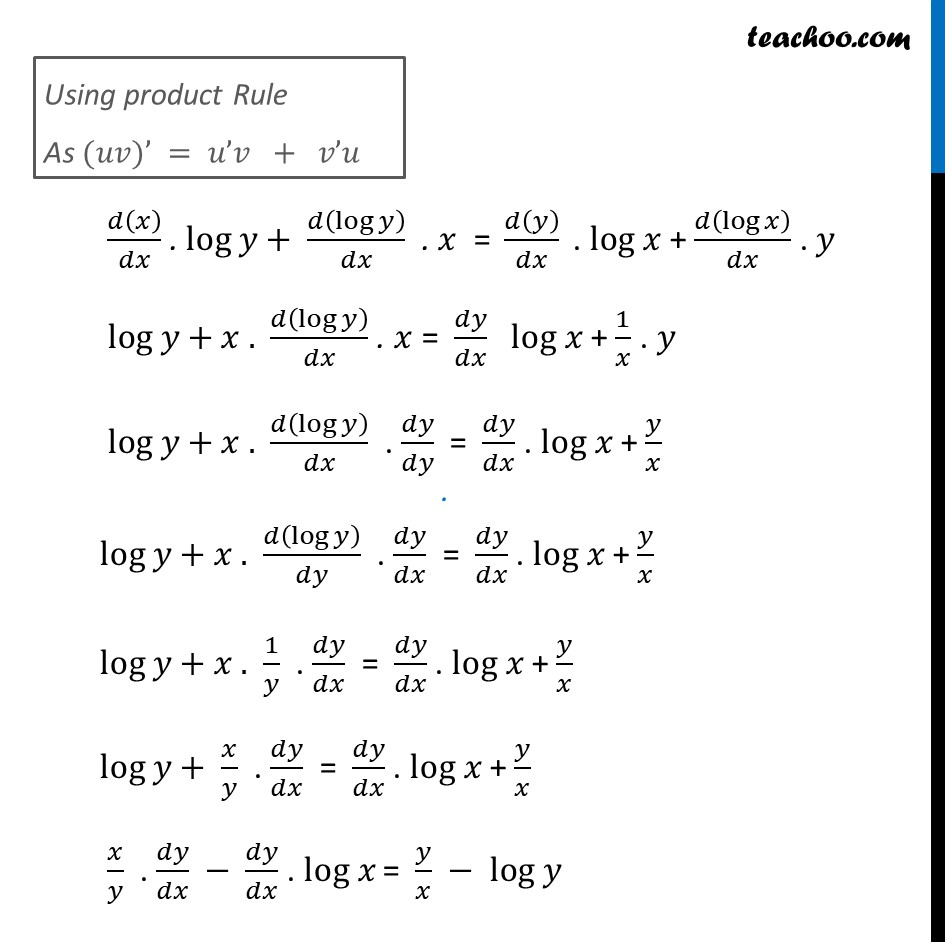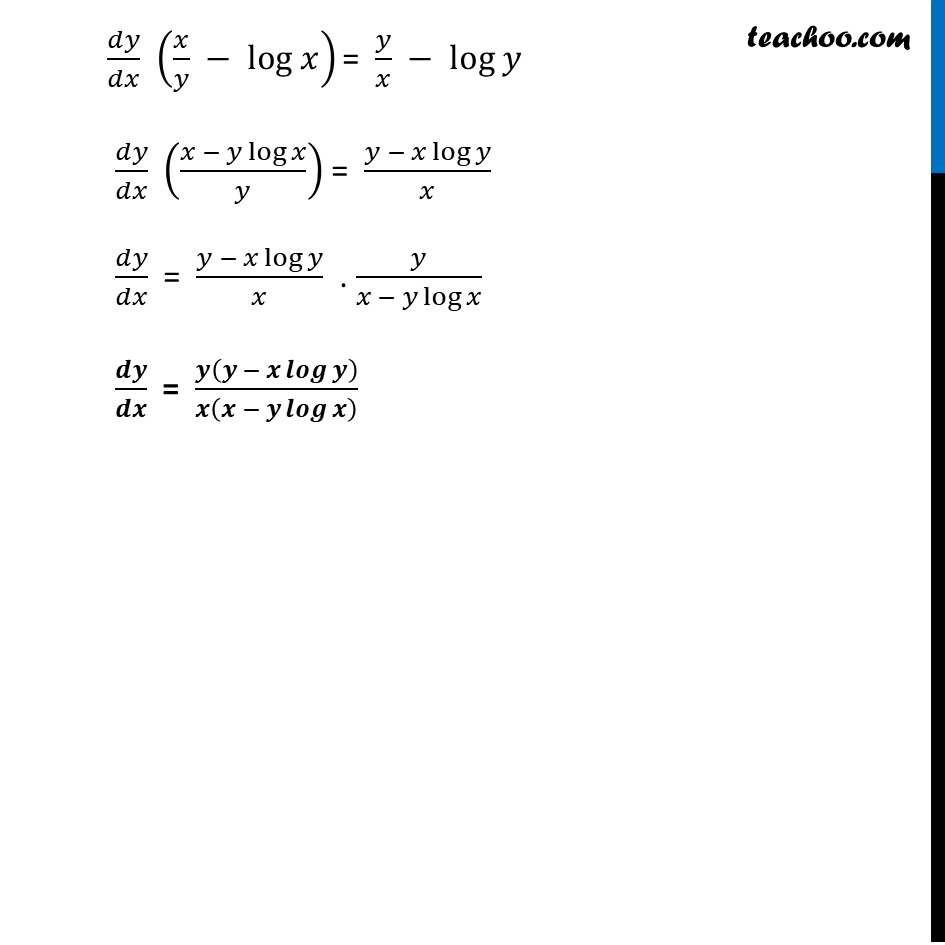Logarithmic Differentiation - Type 1

Chapter 5 Class 12 Continuity and Differentiability
Concept wiseLearn in your speed, with individual attention - Teachoo Maths 1-on-1 Class

### Transcript

Ex 5.5, 13 Find 𝑑𝑦/𝑑𝑥 of the functions in, 𝑦^𝑥 = 𝑥^𝑦 Given, 𝑦^𝑥 = 𝑥^𝑦 Taking log both sides log (𝑦^𝑥 ) = log (𝑥^𝑦 ) 𝑥 . log 𝑦=𝑦.log⁡𝑥 Differentiating both sides 𝑤.𝑟.𝑡.𝑥. (𝑑(𝑥 . log 𝑦))/𝑑𝑥 = 𝑑(𝑦.〖 log〗⁡𝑥 )/𝑑𝑥 (As 𝑙𝑜𝑔⁡(𝑎^𝑏 )=𝑏 . 𝑙𝑜𝑔⁡𝑎) Using product Rule As (𝑢𝑣)’ = 𝑢’𝑣 + 𝑣’𝑢 𝑑(𝑥)/𝑑𝑥 . log 𝑦+ 𝑑(log⁡𝑦 )/𝑑𝑥 . 𝑥 =" " 𝑑(𝑦)/𝑑𝑥 " ". log 𝑥 + 𝑑(log⁡𝑥 )/𝑑𝑥 . 𝑦 log 𝑦+𝑥 . 𝑑(log⁡𝑦 )/𝑑𝑥 . 𝑥 = 𝑑𝑦/𝑑𝑥 log 𝑥 + 1/𝑥 . 𝑦 log 𝑦+𝑥 . 𝑑(log⁡𝑦 )/𝑑𝑥 . 𝑑𝑦/𝑑𝑦 = 𝑑𝑦/𝑑𝑥 . log 𝑥 + 𝑦/𝑥 log 𝑦+𝑥 . 𝑑(log⁡𝑦 )/𝑑𝑦 . 𝑑𝑦/𝑑𝑥 = 𝑑𝑦/𝑑𝑥 . log 𝑥 + 𝑦/𝑥 log 𝑦+𝑥 . 1/𝑦 . 𝑑𝑦/𝑑𝑥 = 𝑑𝑦/𝑑𝑥 . log 𝑥 + 𝑦/𝑥 log 𝑦+ 𝑥/𝑦 . 𝑑𝑦/𝑑𝑥 = 𝑑𝑦/𝑑𝑥 . log 𝑥 + 𝑦/𝑥 𝑥/𝑦 . 𝑑𝑦/𝑑𝑥 − 𝑑𝑦/𝑑𝑥 . log 𝑥 = 𝑦/𝑥 − log 𝑦 𝑑𝑦/𝑑𝑥 (𝑥/𝑦 − log 𝑥) = 𝑦/𝑥 − log 𝑦 𝑑𝑦/𝑑𝑥 ((𝑥 − 𝑦 log⁡𝑥)/𝑦) = (𝑦 − 𝑥 log⁡𝑦)/𝑥 𝑑𝑦/𝑑𝑥 = (𝑦 − 𝑥 log⁡𝑦)/𝑥 . 𝑦/(𝑥 − 𝑦 log⁡𝑥 ) 𝒅𝒚/𝒅𝒙 = 𝒚(𝒚 − 𝒙 𝒍𝒐𝒈⁡𝒚 )/𝒙(𝒙 − 𝒚 𝒍𝒐𝒈⁡𝒙 )